## Explain how to write a quadratic equation given the following three points on the graph (5,31) (3,11) (0,11)

Question

Explain how to write a quadratic equation given the following three points on the graph (5,31) (3,11) (0,11)

in progress 0
7 months 2021-07-17T07:18:13+00:00 1 Answers 9 views 0

1. Given:

The graph of a quadratic function passes through the points (5,31) (3,11) (0,11).

To find:

The equation of the quadratic function.

Solution:

A quadratic function is defined as: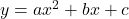…(i)

It is passes through the point (0,11). So, substitute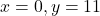in (i).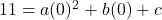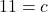Putting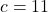in (i), we get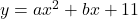…(ii)

The quadratic function passes through the point (5,31). So, substitute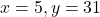in (ii).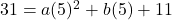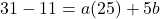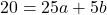Divide both sides by 5.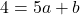…(iii)

The quadratic function passes through the point (3,11). So, substitute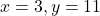in (ii).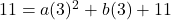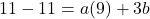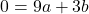Divide both sides by 3.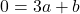…(iv)

Subtracting (iv) from (iii), we get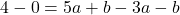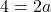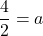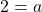Putting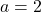in (iv), we get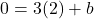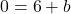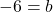Putting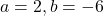in (ii), we get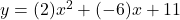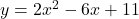Therefore, the required quadratic equation is.